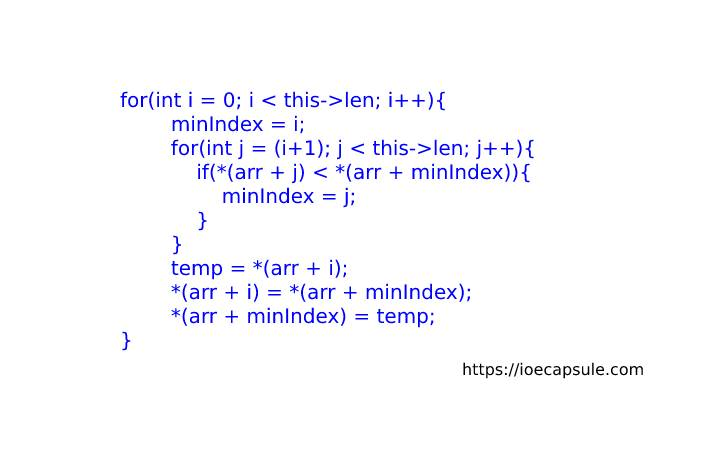### Selection Sort Using C++Algorithms

The selection sort algorithm sorts an array by repeatedly finding the minimum element (considering ascending order) from unsorted part and putting it at the beginning.

In every iteration of selection sort, the minimum element (considering ascending order) from the unsorted subarray is picked and moved to the sorted subarray.

### Selection Sort Using C++

``````
#include<iostream>
using namespace std;

class List{
private:
int* arr;
int len;

public:
List(){
this->arr = NULL;
this->len = 0;
}

void populate(){
do{
cout<<"enter the size:"<<endl;
cin>>this->len;
}while(this->len < 0);

this->arr = new int[this->len];

for(int i = 0; i < this->len; i++){
cout<<"enter "<<i<<"th item"<<endl;
cin>>*(arr+i);
}
}

void selectionSort(){
if(arr == NULL){
cout<<"list is empty !"<<endl;
return;
}
int minIndex, temp;
for(int i = 0; i < this->len; i++){
minIndex = i;
for(int j = (i+1); j < this->len; j++){
if(*(arr + j) < *(arr + minIndex)){
minIndex = j;
}
}
temp = *(arr + i);
*(arr + i) = *(arr + minIndex);
*(arr + minIndex) = temp;
}
}

int length(){
return len;
}

void display(){
if(arr == NULL){
cout<<"list is empty !"<<endl;
return;
}
cout<<"[";
for(int i = 0; i < this->len; i++){
if(i != len-1){
cout<<*(arr+i)<<", ";
}else{
cout<<*(arr+i);
}
}
cout<<"]"<<endl;
}

~List(){
delete arr;
}
};

int main(){
List list;
list.populate();
cout<<"unsorted list:";
list.display();
cout<<"sorted list:";
list.selectionSort();
list.display();
return 0;
}

``````